# Hausdorff series in semigroup rings of rectangular bands

### Full PDFcreative_2023_32_1_49_54

Issue no:

The Hausdorff series provides a solution to the equation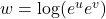given by a recursive formula which can be expressed as nested commutators ofand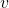.
Evolutions of the Haussdorff series in various algebras and rings has been considered in obtaining a closed form of this formula. We consider the rectangular band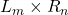determined by the left zero semigroup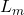and the right zero semigroup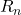of orderand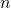, respectively. Let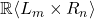be the semigroup ring spanned ontogether with the identity element. We provide a closed form of the formula for solving the equation in.